# rpmfreqmap

Frequency-RPM map for order analysis

## Syntax

``map = rpmfreqmap(x,fs,rpm)``
``map = rpmfreqmap(x,fs,rpm,res)``
``map = rpmfreqmap(___,Name,Value)``
``````[map,freq,rpm,time,res] = rpmfreqmap(___)``````
``rpmfreqmap(___)``

## Description

example

````map = rpmfreqmap(x,fs,rpm)` returns the frequency-RPM map matrix, `map`, that results from performing frequency analysis on the input vector, `x`. `x` is measured at a set `rpm` of rotational speeds expressed in revolutions per minute. `fs` is the sample rate in Hz. Each column of `map` contains root-mean-square (RMS) amplitude estimates of the spectral content present at each value of `rpm`. `rpmfreqmap` uses the short-time Fourier transform to analyze the spectral content of `x`.```

example

````map = rpmfreqmap(x,fs,rpm,res)` specifies the resolution bandwidth of the map in Hz.```

example

````map = rpmfreqmap(___,Name,Value)` specifies options using `Name,Value` pairs in addition to the input arguments in previous syntaxes.```
``````[map,freq,rpm,time,res] = rpmfreqmap(___)``` returns vectors with the frequencies, rotational speeds, and time instants at which the frequency map is computed. It also returns the resolution bandwidth used.```

example

````rpmfreqmap(___)` with no output arguments plots the frequency map as a function of rotational speed and time on an interactive figure. The plot is also known as a Campbell diagram.```

## Examples

collapse all

Create a simulated signal sampled at 600 Hz for 5 seconds. The system that is being tested increases its rotational speed from 10 to 40 revolutions per second during the observation period.

```fs = 600; t1 = 5; t = 0:1/fs:t1; f0 = 10; f1 = 40; rpm = 60*linspace(f0,f1,length(t));```

The signal consists of four harmonically related chirps with orders 1, 0.5, 4, and 6. The order-4 chirp has twice the amplitude of the others. To generate the chirps, use the trapezoidal rule to express the phase as the integral of the rotational speed.

```o1 = 1; o2 = 0.5; o3 = 4; o4 = 6; ph = 2*pi*cumtrapz(rpm/60)/fs; x = [1 1 2 1]*cos([o1 o2 o3 o4]'*ph);```

Visualize the frequency-RPM map of the signal.

`rpmfreqmap(x,fs,rpm)`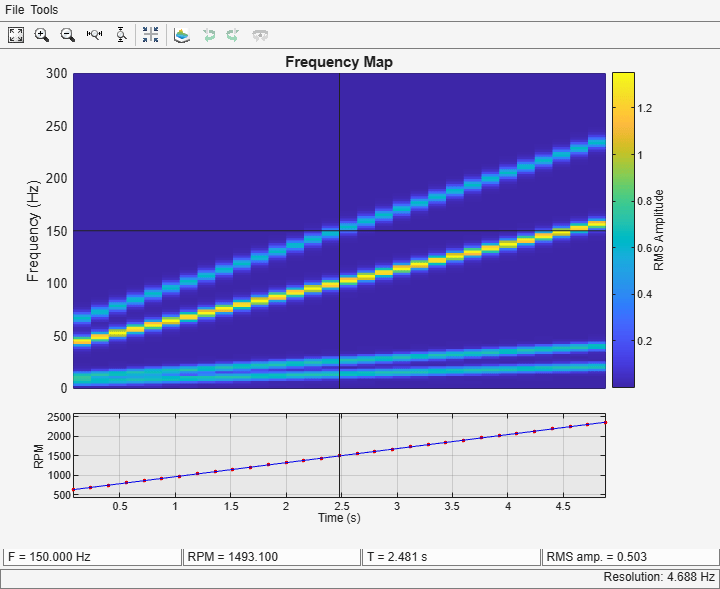Analyze simulated data from an accelerometer placed in the cockpit of a helicopter.

Load the helicopter data. The vibrational measurements, `vib`, are sampled at a rate of 500 Hz for 10 seconds. Inspection of the data reveals that it has a linear trend. Remove the trend to prevent it from degrading the quality of the frequency estimation.

```load('helidata.mat') vib = detrend(vib);```

Plot the nonlinear RPM profile. The rotor runs up until it reaches a maximum rotational speed of about 27,600 revolutions per minute and then coasts down.

```plot(t,rpm) xlabel('Time (s)') ylabel('RPM')```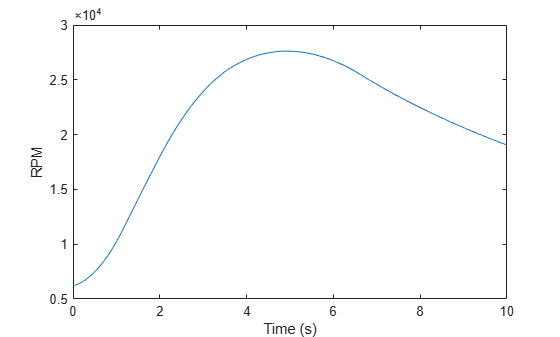Compute the frequency-RPM map. Specify a resolution bandwidth of 2.5 Hz.

`[map,freq,rpmOut,time] = rpmfreqmap(vib,fs,rpm,2.5);`

Visualize the map.

```imagesc(time,freq,map) ax = gca; ax.YDir = 'normal'; xlabel('Time (s)') ylabel('Frequency (Hz)')```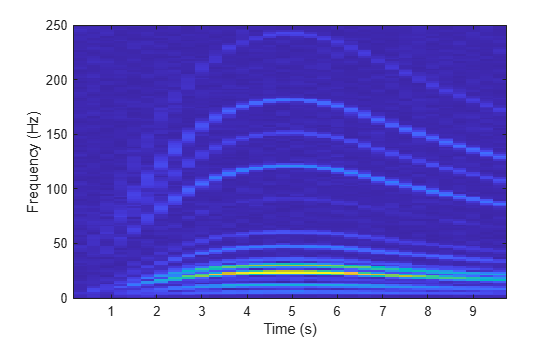Repeat the computation using a finer resolution bandwidth. Plot the map using the built-in functionality of `rpmfreqmap`. The gain in frequency resolution comes at the expense of time resolution.

`rpmfreqmap(vib,fs,rpm,1.5);`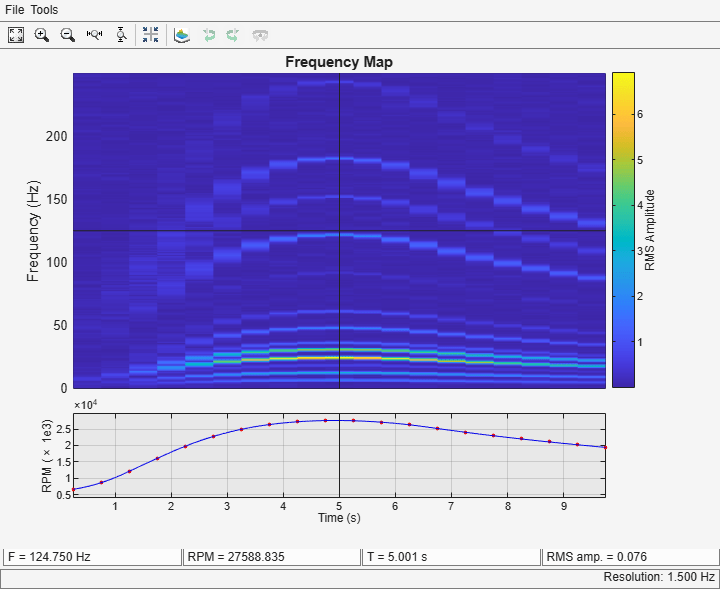Generate a signal that consists of two linear chirps and a quadratic chirp, all sampled at 600 Hz for 15 seconds. The system that produces the signal increases its rotational speed from 10 to 40 revolutions per second during the testing period.

```fs = 600; t1 = 15; t = 0:1/fs:t1; f0 = 10; f1 = 40; rpm = 60*linspace(f0,f1,length(t));```

The linear chirps have orders 1 and 2.5. The component with order 1 has half the amplitude of the other. The quadratic chirp starts at order 6 and returns to this order at the end of the measurement. Its amplitude is 0.8. Create the signal using this information.

```o1 = 1; o2 = 2.5; o6 = 6; x = 0.5*chirp(t,o1*f0,t1,o1*f1)+chirp(t,o2*f0,t1,o2*f1) + ... 0.8*chirp(t,o6*f0,t1,o6*f1,'quadratic');```

Compute the frequency-RPM map of the signal. Use the peak amplitude at each measurement cell. Specify a resolution of 6 Hz. Window the data with a flat top window.

```[map,fr,rp] = rpmfreqmap(x,fs,rpm,6, ... 'Amplitude','peak','Window','flattopwin');```

Draw the frequency-RPM map as a waterfall plot.

```[FR,RP] = meshgrid(fr,rp); waterfall(FR,RP,map') view(-6,60) xlabel('Frequency (Hz)') ylabel('RPM') zlabel('Amplitude')```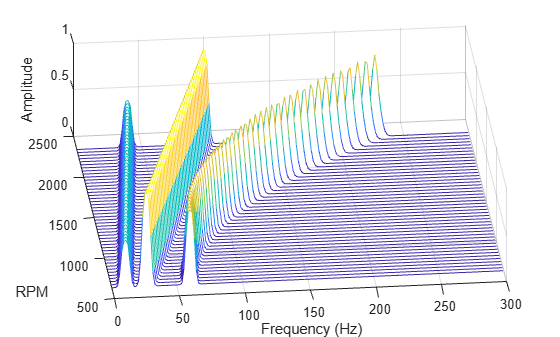Plot an interactive frequency-RPM map by calling `rpmfreqmap` without output arguments.

Load a file containing simulated vibrational data from an accelerometer placed in the cockpit of a helicopter. The data is sampled at a rate of 500 Hz for 10 seconds. Remove the linear trend in the data. Call `rpmfreqmap` to generate an interactive plot of the frequency-RPM map. Specify a frequency resolution of 2 Hz.

```load helidata.mat rpmfreqmap(detrend(vib),fs,rpm,2)```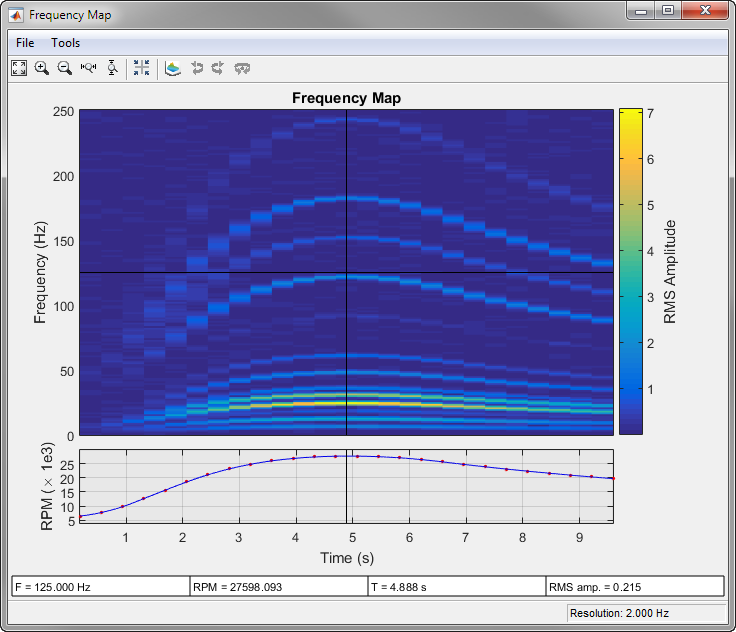Move the crosshair cursors in the figure to determine the RPM and the RMS amplitude at a frequency of 25 Hz after 5 seconds.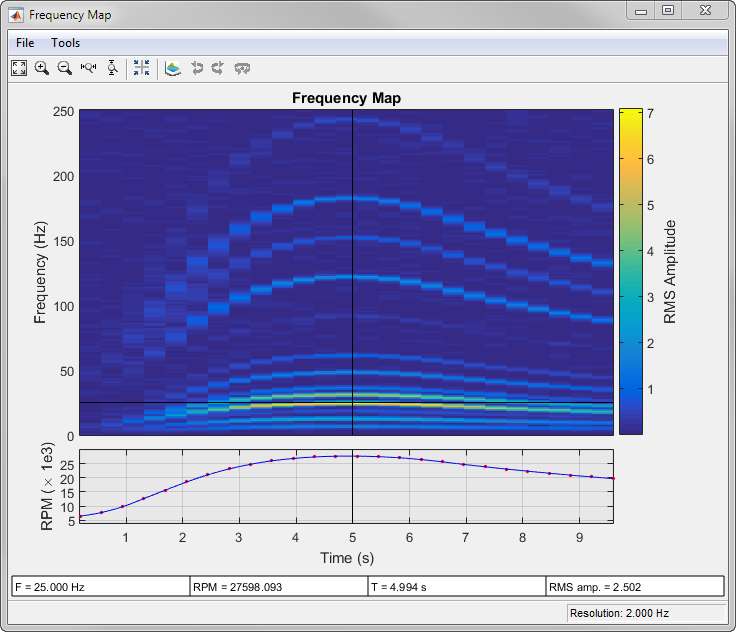Click the buttonin the toolbar to zoom into the time region between 2 and 4 seconds. A panner appears in the bottom plot.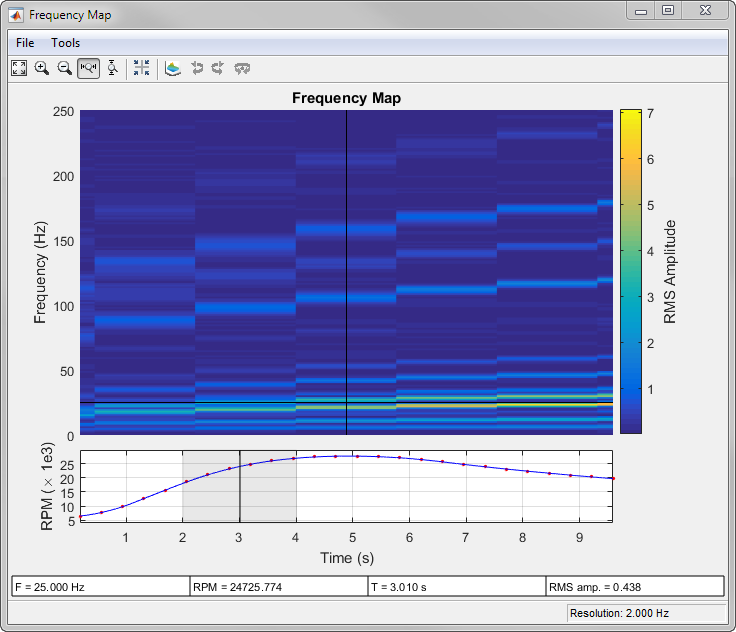Click the button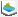in the toolbar to display the frequency-RPM map as a waterfall plot. For improved visibility, rotate the plot clockwise using the button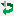three times. Move the panner to the interval between 4 and 6 seconds.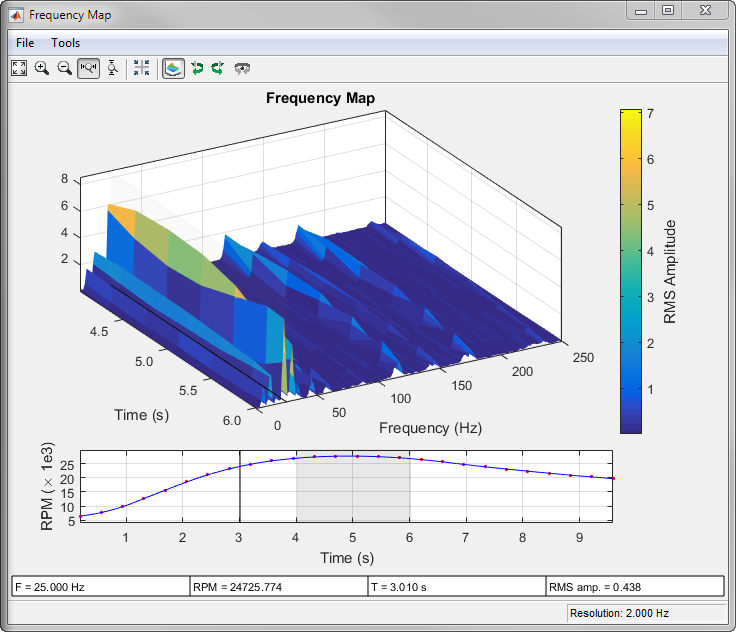## Input Arguments

collapse all

Input signal, specified as a row or column vector.

Example: `cos(pi/4*(0:159))+randn(1,160)` specifies a sinusoid embedded in white Gaussian noise.

Data Types: `double` | `single`

Sample rate, specified as a positive scalar expressed in Hz.

Data Types: `double` | `single`

Rotational speeds, specified as a vector of positive values expressed in revolutions per minute. `rpm` must have the same length as `x`.

• If you have a tachometer pulse signal, use `tachorpm` to extract `rpm` directly.

• If you do not have a tachometer pulse signal, use `rpmtrack` to extract `rpm` from a vibration signal.

Example: `100:10:3000` specifies that a system rotates initially at 100 revolutions per minute and runs up to 3000 revolutions per minute in increments of 10.

Data Types: `double` | `single`

Resolution bandwidth of the frequency-RPM map, specified as a positive scalar. If `res` is not specified, then `rpmfreqmap` sets it to the sample rate divided by 128. If the signal is not long enough, then the function uses the entire signal length to compute a single frequency estimate.

Data Types: `single` | `double`

### Name-Value Pair Arguments

Specify optional comma-separated pairs of `Name,Value` arguments. `Name` is the argument name and `Value` is the corresponding value. `Name` must appear inside quotes. You can specify several name and value pair arguments in any order as `Name1,Value1,...,NameN,ValueN`.

Example: `'Scale','dB','Window','hann'` specifies that the frequency map estimates are to be scaled in decibels and determined using a Hann window.

Frequency-RPM map amplitudes, specified as the comma-separated pair consisting of `'Amplitude'` and one of `'rms'`, `'peak'`, or `'power'`.

• `'rms'` — Returns the root-mean-square amplitude for each estimated frequency.

• `'peak'` — Returns the peak amplitude for each estimated frequency.

• `'power'` — Returns the power level for each estimated frequency.

Overlap percentage between adjoining segments, specified as the comma-separated pair consisting of `'OverlapPercent'` and a scalar from 0 to 100. A value of 0 means that adjoining segments do not overlap. A value of 100 means that adjoining segments are shifted by one sample. A larger overlap percentage produces a smoother map but increases the computation time. See `rpmordermap` for more information.

Data Types: `double` | `single`

Frequency-RPM map scaling, specified as the comma-separated pair consisting of `'Scale'` and either `'linear'` or `'dB'`.

• `'linear'` — Returns a linearly scaled map.

• `'dB'` — Returns a logarithmic map with values expressed in decibels.

Analysis window, specified as the comma-separated pair consisting of `'Window'` and one of these values:

• `'hann'` specifies a Hann window. See `hann` for more details.

• `'chebwin'` specifies a Chebyshev window. Use a cell array to specify a sidelobe attenuation in decibels. The sidelobe attenuation must be greater than 45 dB. If not specified, it defaults to 100 dB. See `chebwin` for more details.

• `'flattopwin'` specifies a flat top window. See `flattopwin` for more details.

• `'hamming'` specifies a Hamming window. See `hamming` for more details.

• `'kaiser'` specifies a Kaiser window. Use a cell array to specify a shape parameter, β. The shape parameter must be a positive scalar. If not specified, it defaults to 0.5. See `kaiser` for more details.

• `'rectwin'` specifies a rectangular window. See `rectwin` for more details.

Example: `'Window','chebwin'` specifies a Chebyshev window with a sidelobe attenuation of 100 dB.

Example: `'Window',{'chebwin',60}` specifies a Chebyshev window with a sidelobe attenuation of 60 dB.

Example: `'Window','kaiser'` specifies a Kaiser window with a shape parameter of 0.5.

Example: `'Window',{'kaiser',1}` specifies a Kaiser window with a shape parameter of 1.

Data Types: `char` | `string` | `cell`

## Output Arguments

collapse all

Frequency-RPM map, returned as a matrix.

Frequencies, returned as a vector.

Rotational speeds, returned as a vector.

Time instants, returned as a vector.

Resolution bandwidth, returned as a scalar.

 Brandt, Anders. Noise and Vibration Analysis: Signal Analysis and Experimental Procedures. Chichester, UK: John Wiley & Sons, 2011.